# Natural Image Statistics and the Visual Cortex

We approach the visual coding in the cortex by considering the statistical properties of natural images. It is reasonable to assume that the visual system is adapted to process the particular kind of input it receives.

A fundamental property of typical visual input is its nonnormality: The outputs of Gabor filters have statistical distibutions that are far from Gaussian. This property is captured by the recently developed model of independent component analysis (ICA).

Consider a typical natural image: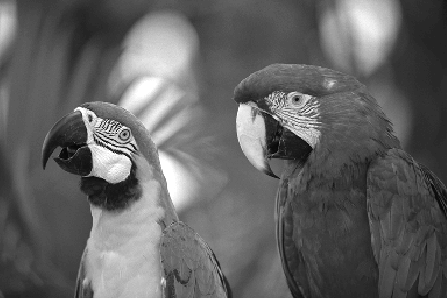When we do ICA on image data, that means we are simply trying to find a representation of the form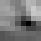= s1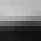+ s2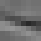+ ... + sk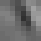such that for any given patch from the image, information about one of the coefficients gives as little information as possible about the others. In other words, they are independent. A fundamental result in the theory of ICA says that when we maximize the independence, we are at the same time maximizing the nongaussianity or the sparseness of the coefficients (components).

Several investigations by different research groups have indicated that such an objective gives basis patches which are localized both in space and in frequency. In fact, the basis patches have the principal properties of the classical receptive fields of simple cells in the visual cortex. Here is an example of such a basis: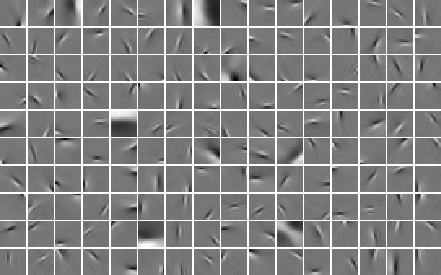It can thus be argued that the functional reason why simple cells resemble Gabor filters is the statistical efficiency of the representation given by independent component analysis.

Our goal is to develop further models of the statistical structure of natural images, and thus to model further properties of the visual cortex.

Patrik Hoyer & Aapo Hyvarinen
March 2001